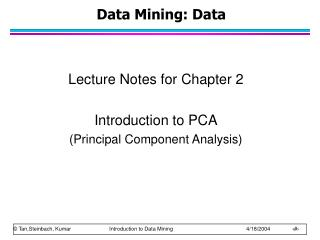DownloadDownload PresentationData Mining: Data

# Data Mining: Data

Download Presentation## Data Mining: Data

- - - - - - - - - - - - - - - - - - - - - - - - - - - E N D - - - - - - - - - - - - - - - - - - - - - - - - - - -
##### Presentation Transcript

1. Data Mining: Data Lecture Notes for Chapter 2 Introduction to PCA (Principal Component Analysis)

2. What is PCA? • Stands for “Principal Component Analysis” • Useful technique in many applications such as face recognition, image compression, finding patterns in data of high dimension • Before introducing this topic, you should know the background knowledge about • Standard deviation • Covariance • Eigenvectors • Eigenvalues (Elementary Linear Algegra)

3. What is PCA? • “It is a way of identifying patterns in data and expressing the data in such a way as to highlight their similarities and differences” • PCA is a powerful tool for analyzing data • Finding the patterns in the data (Feature extraction)— as in the name “Principal Component” means major or maximum information • Reducing the number of dimensions without much loss of information (data reduction, noise rejection, visualization, data compression etc.)

4. Application of PCA • Bivariate of Data set

5. Tutorial by Example • Step1: Get some data

6. Tutorial by Example • Step2: Make a data set whose mean is zero • Compute the mean and std, Then subtract the mean from each of data dimensions

7. Tutorial by Example

8. Tutorial by Example • Step3: Calculate the covariance matrix (see PCATutorial.pdf) Since the data is 2 dim, the covariance matrix will be 2x2 • What to notice?

9. Tutorial by Example • Step4: Calculate the eigenvectors and eigenvalues of the covariance matrix

10. Tutorial by Example

11. Tutorial by Example • Step5: Choosing components and forming a feature vector • The eigenvector with the highest eigenvalue is the principle component of the data set • The principle component from the example • You can decide to ignore the components of lesser significance, you do lose some information • If the eigenvalues are small, you don’t lose much • If you leave out some components, the final data set will have less dimensions (features) than the original

12. Tutorial by Example • Then after ordering the eigenvectors by eigenvalues (highest to lowest), this can form a feature vector FeatureVector = (eig1 eig2 eig3 … eign) • From this example, we have two eigenvectors • So we have two chioces • Form a featuer vector with both of the eigenvectors • Leave out smaller, less significant component and only have a single column

13. Tutorial by Example • Step6 : Deriving the new data set

14. Tutorial by Example

15. Tutorial by Example